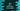# Java program to print the ASCII value of an integer## Java program to print ASCII value of an integer :

American Standard Code for Information Interchange or ASCII code links an integer value to a symbol like letter, digit , special character etc. In ASCII 7 bits are used to represent a character. From 0 to 127, each number can be represent by a character. In this tutorial, we will learn how to print ASCII value of an integer.

### Java Program :

``````import java.util.Scanner;

public class Main {

/**
* Utility functions
*/
static void println(String string) {
System.out.println(string);
}

static void print(String string) {
System.out.print(string);
}

public static void main(String[] args) {
Scanner sc = new Scanner(System.in);

println("********** Java program to convert ASCII to Character **********");
println("");

int ascii;

println("Enter a ASCII value within 0 to 127 :");

ascii = sc.nextInt();

if (ascii > 127) {
println("Please enter a valid input !!");
} else {
//convert the integer value to character
char asciiChar = (char) ascii;

println("ASCII value of " + ascii + " is " + asciiChar);
}
}
}
``````

### Sample Output:

``````Enter a ASCII value within 0 to 127 :
124
ASCII value of 124 is |

Enter a ASCII value within 0 to 127 :
82
ASCII value of 82 is R

Enter a ASCII value within 0 to 127 :
92
ASCII value of 92 is \

Enter a ASCII value within 0 to 127 :
120
ASCII value of 120 is x
``````